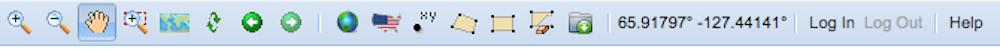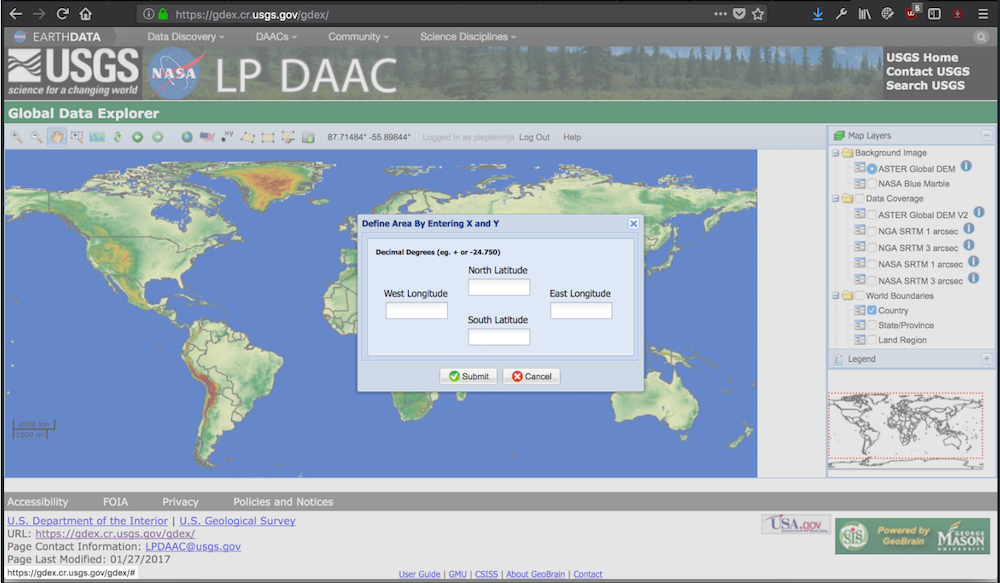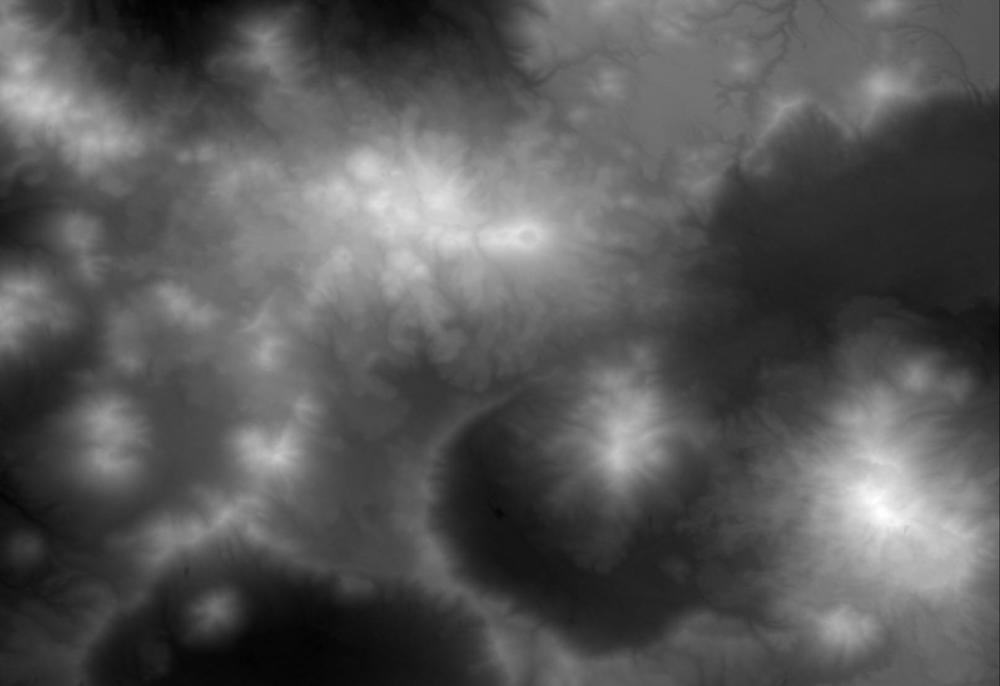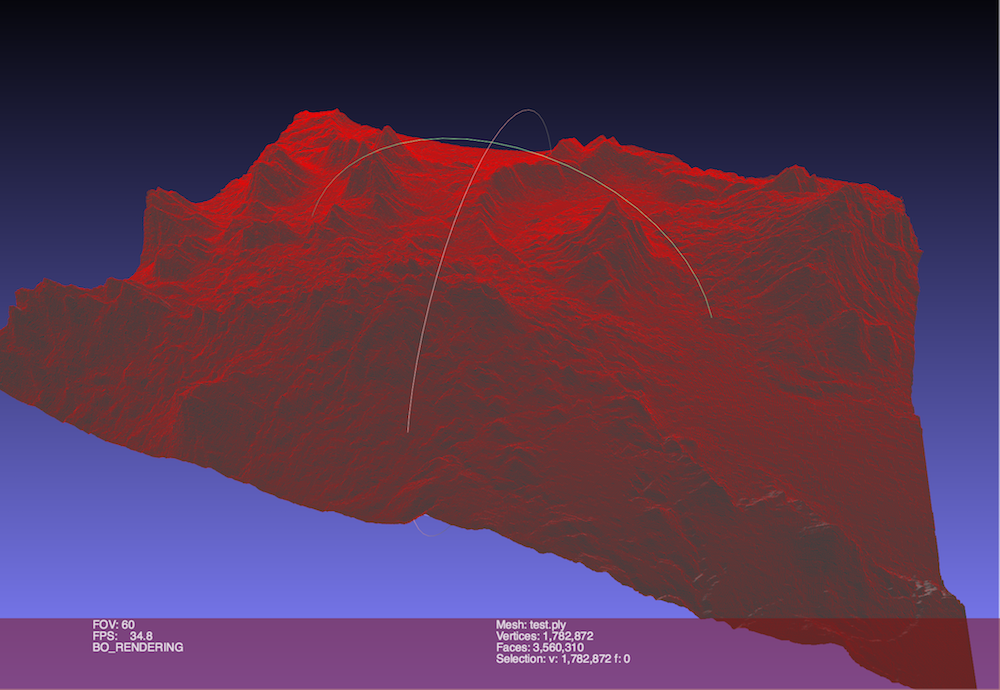## Getting 3D Models of the Earth's Surface

Ever wanted a 3D model of some specific terrain on Earth? Well I have. Good thing NASA puts that out for everyone to see!

In 1999 NASA launched the Advanced Spaceborne Thermal Emission and Reflection Radiometer, quite the mouth-full, otherwise known as ASTER. This satellite’s data is used to create Global Digital Elevation Models, or DEMs. Ever sense April 2016 NASA made this data free for the public! All you have to do is follow these steps to make a 3D .ply, which is the Stanford polygon file format, of anywhere on the planet!

First, travel over to the USGS Data Explorer

You need to log in or make an account in that navbar area:Once you’ve logged in, then you can define the area that you want to get a model of. You can do this by dragging a box or defining latitude and longitude bounds:Select Download Data for defined Area. First, select JPEG as the file type. Congrats, you should see a black and white JPEG! … download it. We need to install the GDAL libraries so that we can manipulate this data. For me this was as easy as:

brew install gdal


If you’re on macOS and you don’t have homebrew, really what are you doing? Anyway, The final this we want to do is convert this JPEG into a 3D file. I downloaded the Ojos del Salado Mountain Range:Essentially we want to convert the black and white areas to heights. I use this simple python script to accomplish this:

#!/usr/bin/python

import sys
import numpy as np
from osgeo import gdal

def write_ply(filename, coordinates, triangles, binary=True):
template = "ply\n"
if binary:
template += "format binary_" + sys.byteorder + "_endian 1.0\n"
else:
template += "format ascii 1.0\n"
template += """element vertex {nvertices:n}
property float x
property float y
property float z
element face {nfaces:n}
property list int int vertex_index
"""

context = {
"nvertices": len(coordinates),
"nfaces": len(triangles)
}

if binary:
with  open(filename,'wb') as outfile:
outfile.write(template.format(**context))
coordinates = np.array(coordinates, dtype="float32")
coordinates.tofile(outfile)

triangles = np.hstack((np.ones([len(triangles),1], dtype="int") * 3,
triangles))
triangles = np.array(triangles, dtype="int32")
triangles.tofile(outfile)
else:
with  open(filename,'w') as outfile:
outfile.write(template.format(**context))
np.savetxt(outfile, coordinates, fmt="%.3f")
np.savetxt(outfile, triangles, fmt="3 %i %i %i")

raster = gdal.Open(filename)
print '== Detected =='
print 'X Size: ' + str(raster.RasterXSize)
print raster.RasterXSize
print 'Y Size: ' + str(raster.RasterYSize)
print raster.RasterYSize
return raster

def createvertexarray(raster):
transform = raster.GetGeoTransform()
width = raster.RasterXSize
height = raster.RasterYSize
x = np.arange(0, width) * transform + transform
y = np.arange(0, height) * transform + transform
xx, yy = np.meshgrid(x, y)
vertices = np.vstack((xx,yy,zz)).reshape([3, -1]).transpose()
return vertices

def createindexarray(raster):
width = raster.RasterXSize
height = raster.RasterYSize

ai = np.arange(0, width - 1)
aj = np.arange(0, height - 1)
aii, ajj = np.meshgrid(ai, aj)
a = aii + ajj * width
a = a.flatten()

tria = np.vstack((a, a + width, a + width + 1, a, a + width + 1, a + 1))
tria = np.transpose(tria).reshape([-1, 3])
return tria

def main(argv):
inputfile = argv
outputfile = argv

vertices = createvertexarray(raster)
triangles = createindexarray(raster)

write_ply(outputfile, vertices, triangles, binary=True)

if __name__ == "__main__":
main(sys.argv[1:])



My results: